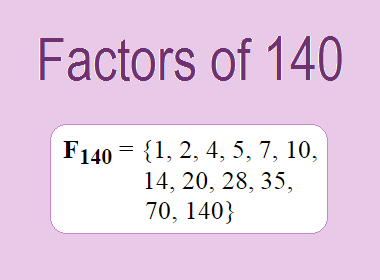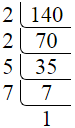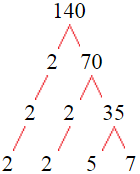# Factors of 140The factors of 140 are 1, 2, 4, 5, 7, 10, 14, 20, 28, 35, 70, and 140 i.e. F140 = {1, 2, 4, 5, 7, 10, 14, 20, 28, 35, 70, 140}. The factors of 140 are all the numbers that can divide 140 without leaving a remainder.

We can check if these numbers are factors of 140 by dividing 140 by each of them. If the result is a whole number, then the number is a factor of 140. Let's do this for each of the numbers listed above:

·        1 is a factor of 140 because 140 divided by 1 is 140.

·        2 is a factor of 140 because 140 divided by 2 is 70.

·        4 is a factor of 140 because 140 divided by 4 is 35.

·        5 is a factor of 140 because 140 divided by 5 is 28.

·        7 is a factor of 140 because 140 divided by 7 is 20.

·        10 is a factor of 140 because 140 divided by 10 is 14.

·        14 is a factor of 140 because 140 divided by 14 is 10.

·        20 is a factor of 140 because 140 divided by 20 is 7.

·        28 is a factor of 140 because 140 divided by 28 is 5.

·        35 is a factor of 140 because 140 divided by 35 is 4.

·        70 is a factor of 140 because 140 divided by 70 is 2.

·        140 is a factor of 140 because 140 divided by 140 is 1.

## How to Find Factors of 140?

1 and the number itself are the factors of every number. So, 1 and 140 are two factors of 140. To find the other factors of 140, we can start by dividing 140 by the numbers between 1 and 140. If we divide 140 by 2, we get a remainder of 0. Therefore, 2 is a factor of 140. If we divide 140 by 3, we get a remainder of 2. Therefore, 3 is not a factor of 140.

Next, we can check if 4 is a factor of 140. If we divide 140 by 4, we get a remainder of 0. Therefore, 4 is a factor of 140. We can continue this process for all the possible factors of 140.

Through this process, we can find that the factors of 140 are 1, 2, 4, 5, 7, 10, 14, 20, 28, 35, 70, and 140. These are the only numbers that can divide 140 without leaving a remainder.

********************

********************

## Properties of the Factors of 140

The factors of 140 have some interesting properties. One of the properties is that the sum of the factors of 140 is equal to 336. We can see this by adding all the factors of 140 together:

1 + 2 + 4 + 5 + 7 + 10 + 14 + 20 + 28 + 35 + 70 + 140 = 336

Another property of the factors of 140 is that the prime factors of 140 are 2, 5, and 7 only.

## Applications of the Factors of 140

The factors of 140 have several applications in mathematics. One of the applications is in finding the highest common factor (HCF) of two or more numbers. The HCF is the largest factor that two or more numbers have in common. For example, to find the HCF of 140 and 84, we need to find the factors of both numbers and identify the largest factor they have in common. The factors of 140 are 1, 2, 4, 5, 7, 10, 14, 20, 28, 35, 70, and 140. The factors of 84 are 1, 2, 3, 4, 6, 7, 12, 14, 21, 28, 42, and 84. The largest factor that they have in common is 28. Therefore, the HCF of 140 and 84 is 28.

Another application of the factors of 140 is in prime factorization. Prime factorization is the process of expressing a number as the product of its prime factors. The prime factors of 140 are 2, 5, and 7, since these are the only prime numbers that can divide 140 without leaving a remainder. Therefore, we can express 140 as:

140 = 2 × 2 × 5 × 7

We can do prime factorization by division and factor tree method also. Here is the prime factorization of 140 by division method,140 = 2 × 2 × 5 × 7

Here is the prime factorization of 140 by the factor tree method,140 = 2 × 2 × 5 × 7

## Conclusion

The factors of 140 are the numbers that can divide 140 without leaving a remainder. The factors of 140 are 1, 2, 4, 5, 7, 10, 14, 20, 28, 35, 70, and 140. The factors of 140 have some interesting properties, such as having a sum of 336. The factors of 140 have several applications in mathematics, such as finding the highest common factor and prime factorization.